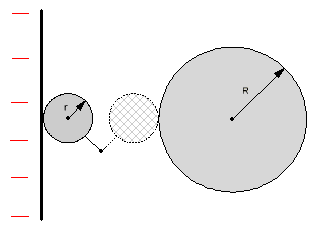Research website of Vyacheslav Gorchilin
2015-01-31
Free energy
In recent times, have significantly increased interest in the surrounding free or "free energy". Actively developed devices receiving and transforming it into forms familiar to us: light, heat, electricity. In this work, we want to offer the theoretical part of the research, which will allow developers to more deeply understand the processes. At the same time, we postrema not extend their calculations beyond the school physics course!
Where does the energy come from?

On one famous forum for several years had discussed such a problem: if a single charged capacitor connected in parallel with the others discharged, in theory, the total potential energy of the two capacitors will be two times less. The following question was asked: where does the energy come from?

The solution proposed is different from the version that the law of conservation of energy is more important than the charge conservation law to the circuit of the capacitors was considered by the internal resistance, on Negev which consumes a portion of energy. The last solution, by the way, is the surest.

But the conditions of the problem can be slightly changed, for example, in the circuit between the two capacitors in series to add resistance and small inductance, such that the internal resistance of the energy spent is less. Or, instead of capacitors to take two identical solitary capacity — balls, for example, charge one with, one without. Then, the internal resistance evaporate by themselves, and the question remains the same: what happens to the energy when connecting two such balls? It is obvious that to resolve the apparent paradox of "dig" deeper need.

The inverse problem

Suppose we have: a source of charge, a small sphere of radius rand a radius R. Both balls are secluded capacity, and R>>r. Let's start to transfer charge to a small ball from a source to a large ball (Fig 1).Fig. 1. The transfer charge from their source to a large ball with small ball

At the beginning of the small ball is charged by the source charge $$q$$ and the following energy: $W_{r} = \frac {q^2}{2C_{r}}$ where: $$C_{r}$$ — the capacity of small ball. The formula to determine the capacity of this solitary globe: $C_{r} = 4\pi \varepsilon \varepsilon_{0}r$ where $$\varepsilon$$ — dielectric permittivity, $$\varepsilon_{0}$$ is the absolute dielectric constant equal to $$8.86\cdot 10^{-12} \; \frac {f} {m}$$.

Next, a small balloon is connected with a large and almost gives him all his power, as the law of conservation of charge: $$q=q_{1} + Q_{1}$$, where $$q_{1}$$ and $$Q_{1}$$, respectively, of charges to small and large balls as they connect. Since $$q_{1}=C_{r}U_{1}$$ and $$Q_{1}=C_{r}U_{1}$$ and $$C_{R} \gg C_{r}$$ , then $$Q_{1} \gg q_{1}$$. We note only that $$U_{1}$$ is the voltage at the United balls, and $$C_{R}$$ is the capacity of a large ball, which is given by: $C_{R} = 4\pi \varepsilon \varepsilon_{0}R$ where: $$R$$ is the radius of the big ball.

This becomes more clear if we consider the law of charge conservation, the sum of a certain number of electrons: how many of them were, so much about the money and left.

The energy of the large ball after the charge transfer becomes equal to: $W_{R1} = \frac {Q_{1}^{2}} {2C_{R}}$ or approximately: $W_{R1} \approx \frac {q^{2}} {2C_{R}}$

It is easy to calculate that after a second operation to transfer the charge on the big ball will be about double, and energy — four times: $W_{R2} \approx \frac {(2q)^{2}} {2C_{R}} = 4 \frac {q^{2}} {2C_{R}}$

It is clearly seen that the energy of a large ball in $$n$$-dimensional step will be equal to: $W_{Rn} \approx n^{2} \frac {q^{2}} {2C_{R}}, \qquad (1.1)$ of course, while the condition $$R \gg r \cdot n$$.

If the work performed each time the charge transfer, denoted by $$A$$, then $$n$$ shifts the total elapsed the work will be this: $$A_{n}=A \cdot n$$. As you can see, it is possible to select such experimental conditions, under which via a number $$n$$ shifts the potential energy of the big ball will be spent more mechanical: $W_{Rn} > A_{n}. \qquad (1.2)$

Since we have set the inverse problem, and the question formulated in the beginning, will also sound different: where does the energy come from? Will reply later, but for now remember electrostatic machine  familiar to us all from the lessons of physics — there is such a principle: the role of source of charge perform rotating discs, the role of small — capacity plate, which is removed from charge, and large capacity is the Leyden jar.

Note. The observant will note that the calculations were not taken into account the Coulomb force. But, in contrast to $$W_{Rn}$$, which is proportional to the square of the $$n$$, the Coulomb force will grow only in proportion to $$n$$, so the qualitative conclusion is not affected.

Another way to increase efficiency due to charge transfer you can read here.